Journal of Accountancy, August 2014 Excel Reader Challenge Summary of Reader Solutions by J. Carlton Collins  Congratulations to Amir Melamed for submitting the best solution to the August 2014 Reader Challenge. Like many other readers, Amir used the Excel Solver approach, but Amir's solution bettered them all to derive a maximum portfolio of \$70,184, as follows: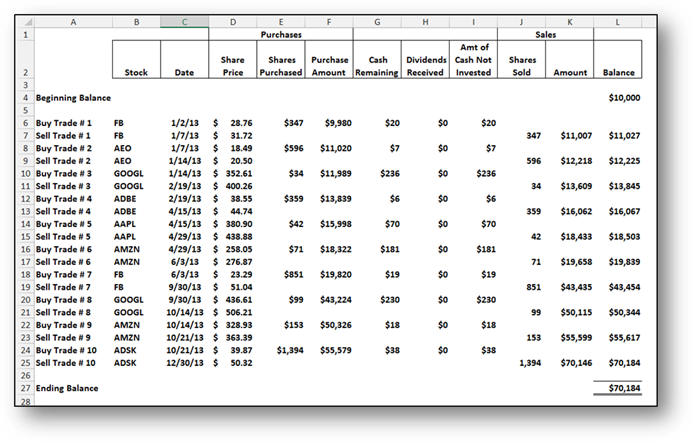You can download Amir’s solution at www.CarltonCollins.com/amir.xlsx. Many readers, including myself, had trouble using Excel’s Solver because this tool is limited, as follows: In Excel Solver, the number of variables is limited to 200, and the number of constraints you can apply to non-decision variables is limited to approximately 100 (depending on whether you use a linear or nonlinear model) and the specific configuration of the constraints. I got around these limitations by dividing my solution up into 4 solver calculations, each solving one quarter of the entire problem, and then combining the results.  Amir ingeniously got around this problem by creating calculations that simplified the problem into a 52 week numbers problem, expressed as integers. (In contrast, I solved for both the ticker and buy/sell date combinations, which resulted in more much computing power than Excel Solver could handle. Amir used calculations to reduce the ticker and date combinations down to just 52 weeks, and Solver was able to handle this simplified approach.) You can see where Amir accomplished this in the screenshot below – he calculated the weekly returns in columns M thru V, and then assigned a week number to each week in column Z.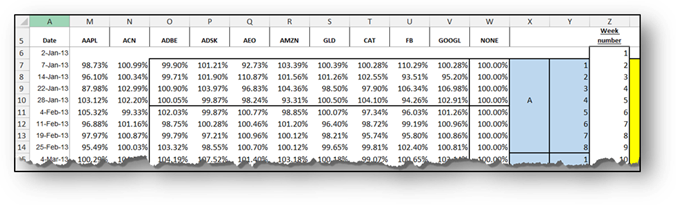Thereafter, he used Solver to identify the specific 10 combinations of the 52 weeks that would derive the top solution. The simplicity of this “week number” approach can be seen in the following screenshot of Amir’s Solver Parameters.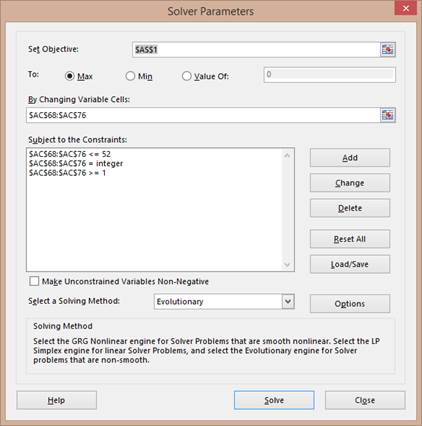Amir’s Objective cell (AS1 in this example), was simply the product of the 10 weekly returns flushed out by his calculations – Solver then calculated the many different combinations of these weekly returns to maximize this amount. Amir’s first and third constraints listed above (AC68:AC76<=52 and AC68:AC76>=1) simply limited each of the ten week digits flushed out by his calculations to the digits 1 to 52, as pictured in yellow below.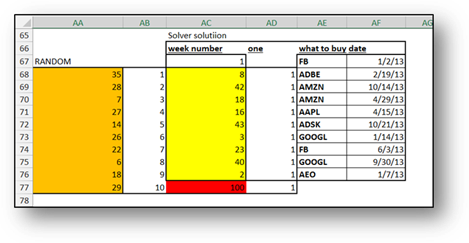Amir’s second constraint listed above (AC68:AC76=integer) limited these yellow cells to integers, so Solver would not attempt to solver for fractional amounts. A Few Learning Points I learned from this challenge that Excel’s solver is limited, I was not aware of these specific limitations, although I did understand that billions of calculations can overwhelm Solver. Further, Amir’s solution helped me understand that Solver works best when you can reduce your problem down to a simple series of integers. I also learned that if you reached the limit of 100 constraints, you may be able to overcome this obstacle by using the following steps: 1.     If you have constraint cells containing simple formulas, such as =A1 (where A1 is a decision variable), then these cells count against the limit of 100; but if you apply the constraint directly to the decision variable cells themselves, it won't. 2.      If a constraint involves two or more decision variables, it might be helpful to place individual limits on these variables instead. 3.     If you have formula based constraints calculated into your worksheet, whose values depend on the decision variables, then Solver treats them against the constraint limit, even if they are decision variables. In this case, you should replace these formula based constraints with individual cell limits, if possible. 4.     If all else fails, an excellent option may be to upgrade to Frontline's Premium Solver Pro which has a limit of 1,000 constraints for linear problems, and 250 constraints for nonlinear problems (apart from bounds on the variables and integer constraints), and it can solve problems faster than Excel Solver. In addition, Frontline’s Large-Scale GRG Solver handles problems with thousands of constraints, in addition to bounds on the variables. You can download a free 15 day trial of these Excel add-in solutions at www.solver.com, as pictured below.I downloaded Frontline’s Premium Solver Pro and added it in to Excel and then used this solution, here is the solution’s ribbon toolbar.I was particularly pleased that Frontline’s Premium Solver Pro automatically detected and picked up the parameter settings from Excel Solver, so I did not have to re-create them from scratch. Recap I had always visualized this challenge as a problem for Excel Solver, but some readers took different approaches to solving this problem, a few of which are briefly mentioned below: 1.      Amir (\$70,184) – As discussed above, Amir used the Solver approach to derive a maximum portfolio of \$70,184. 2.      Berry (\$66,622) – Berry’s solution utilizes a series of MAX functions to flush out the maximum gains for each holding period, and then the MATCH function to extract the corresponding date and ticker information for those MAX gains. 3.      Isai (\$64,784) – Isia used an Array Formula approach to derive a maximum portfolio value of \$64,784. 4.      Jessica (\$62,149) – Jessica calculated the XIRR for each weekly trade for all stocks, and then extracted the highest 10 trades. 5.      Peter (\$41,224) – Peter used a LOOKUP, Formula, Sorting and Filter approach to derive a maximum portfolio of \$41,224. 6.      Richard (\$32,804) – Richard’s solution derived a maximum portfolio value of \$32,804 by calculating the differences between the MIN and MAX prices for each stock, and then eyeballing (apparently) the results to pluck out the top trades.  A summary of the trades recommended by some of the readers is shown below.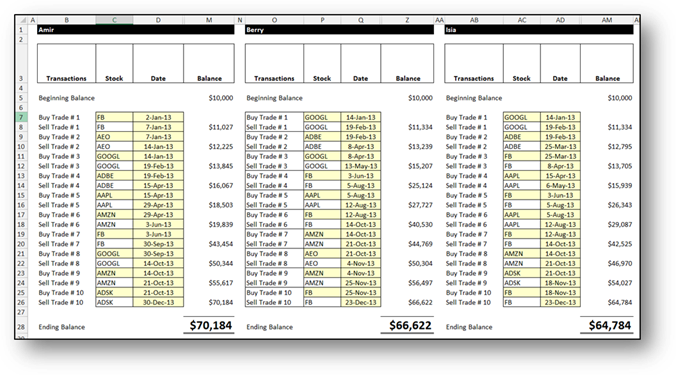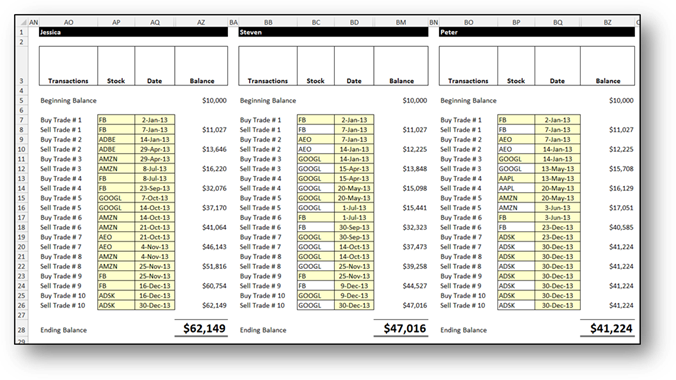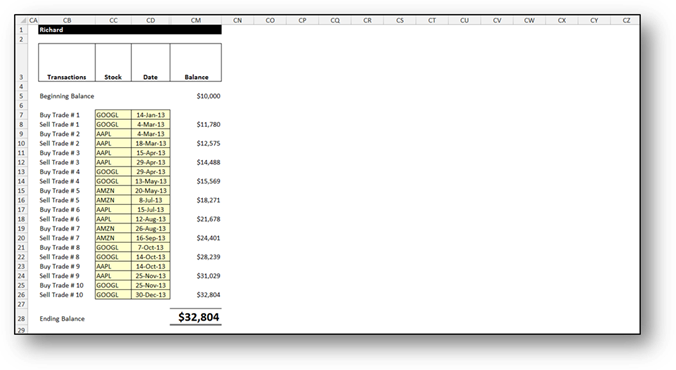Many readers indicated that they struggled with this challenge and ultimately, many of them simply gave up. Kudos to those readers who accepted the challenge, and put forth the effort to submit a solution – you represent the cream of the crop. Congratulations.